## 5.4 Open‑loop pitch analysis

26.1903GPPAdaptive Multi-Rate - Wideband (AMR-WB) speech codecSpeech codec speech processing functionsTranscoding functionsTS

Depending on the mode, open-loop pitch analysis is performed once per frame (each 10 ms) or twice per frame (each 10 ms) to find two estimates of the pitch lag in each frame. This is done in order to simplify the pitch analysis and confine the closed loop pitch search to a small number of lags around the open-loop estimated lags.

Open-loop pitch estimation is based on the weighted speech signal which is obtained by filtering the input speech signal through the weighting filter, where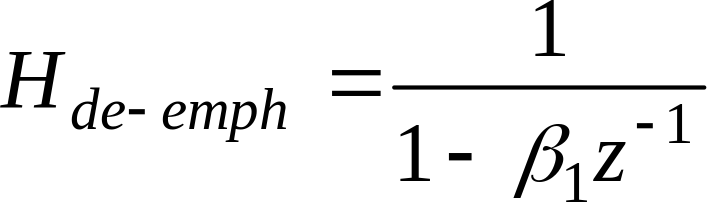and 1=0.68. That is, in a subframe of size L, the weighted speech is given by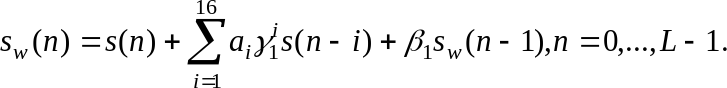( 25 )

The open-loop pitch analysis is performed to a signal decimated by two. The decimated signal is obtained by filtering through a fourth order FIR filterand then downsampling the output by two to obtain the signal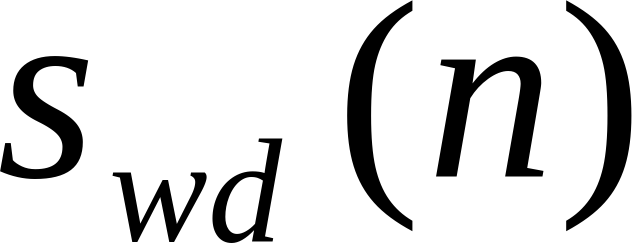.

### 5.4.1 6.60 kbit/s mode

Open-loop pitch analysis is performed once per frame (every 20 ms) to find an estimate of the pitch lag in each frame.

The open-loop pitch analysis is performed as follows. First, the correlation of decimated weighted speech is determined for each pitch lag value d by: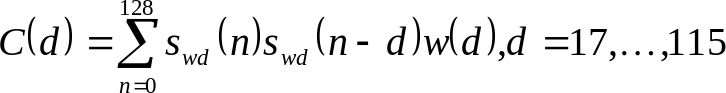, ( 26 )

where w(d) is a weighting function. The estimated pitch-lag is the delay that maximises the weighted correlation function C(d). The weighting emphasises lower pitch lag values reducing the likelihood of selecting a multiple of the correct delay. The weighting function consists of two parts: a low pitch lag emphasis function, wl(d), and a previous frame lag neighbouring emphasis function, wn(d):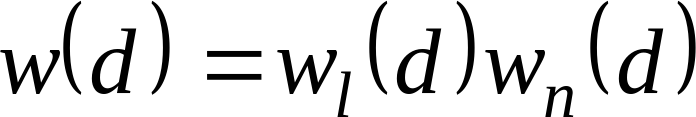. ( 27 )

The low pitch lag emphasis function is a given by: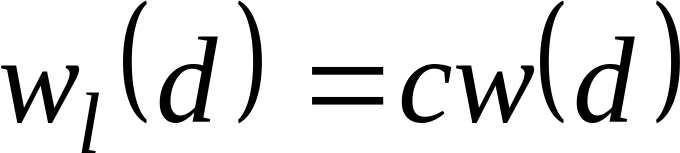( 28 )

where cw(d) is defined by a table in the fixed point computational description. The previous frame lag neighbouring emphasis function depends on the pitch lag of previous speech frames: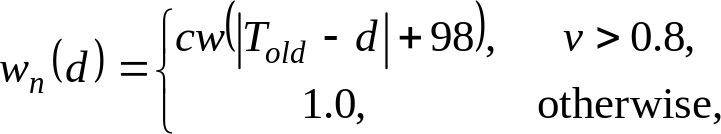( 29 )

where Told is the median filtered pitch lag of 5 previous voiced speech half-frames and v is an adaptive parameter. If the frame is classified as voiced by having the open-loop gain g>0.6, then the v-value is set to 1.0 for the next frame. Otherwise, the v-value is updated by v=0.9v. The open loop gain is given by: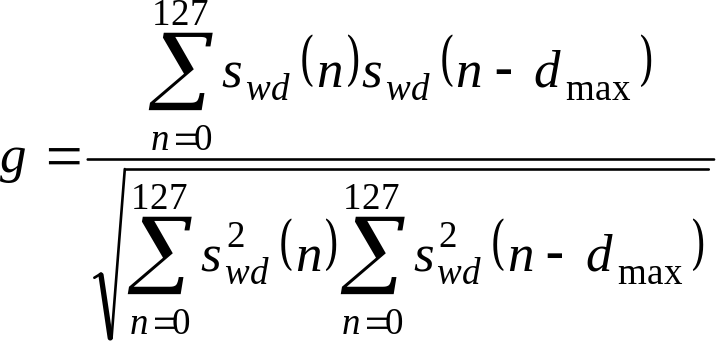( 30 )

where dmax is the pitch delay that maximizes C(d). The median filter is updated only during voiced speech frames. The weighting depends on the reliability of the old pitch lags. If previous frames have contained unvoiced speech or silence, the weighting is attenuated through the parameter v.

### 5.4.2 8.85, 12.65, 14.25, 15.85, 18.25, 19.85, 23.05 and 23.85 kbit/s modes

Open-loop pitch analysis is performed twice per frame (every 10 ms) to find two estimates of the pitch lag in each frame.

The open-loop pitch analysis is performed as follows. First, the correlation of decimated weighted speech is determined for each pitch lag value d by: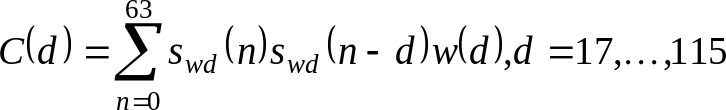, ( 31 )

where w(d) is a weighting function. The estimated pitch-lag is the delay that maximises the weighted correlation function C(d). The weighting emphasises lower pitch lag values reducing the likelihood of selecting a multiple of the correct delay. The weighting function consists of two parts: a low pitch lag emphasis function, wl(d), and a previous frame lag neighbouring emphasis function, wn(d):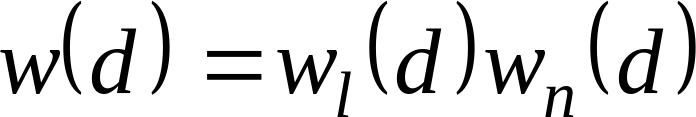. ( 32 )

The low pitch lag emphasis function is given by: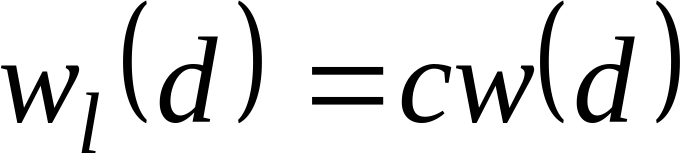( 33 )

where cw(d) is defined by a table in the fixed point computational description. The previous frame lag neighbouring emphasis function depends on the pitch lag of previous speech frames: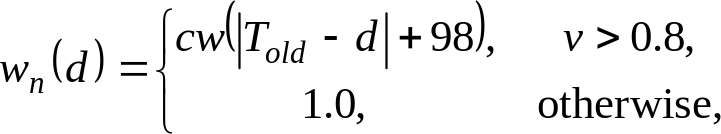( 34)

where Told is the median filtered pitch lag of 5 previous voiced speech half-frames and v is an adaptive parameter. If the frame is classified as voiced by having the open-loop gain g>0.6, then the v-value is set to 1.0 for the next frame. Otherwise, the v-value is updated by v=0.9v. The open loop gain is given by: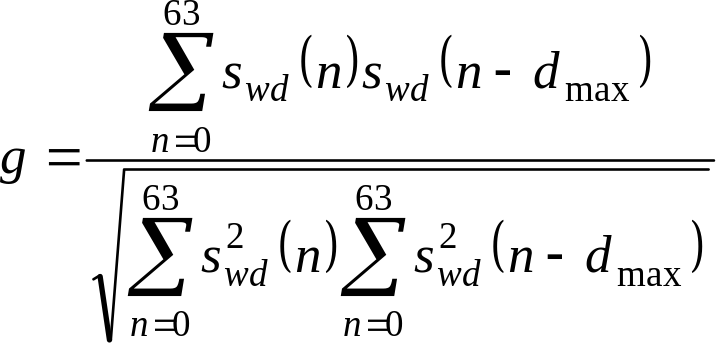( 35)

where dmax is the pitch delay that maximizes C(d). The median filter is updated only during voiced speech frames. The weighting depends on the reliability of the old pitch lags. If previous frames have contained unvoiced speech or silence, the weighting is attenuated through the parameter v.